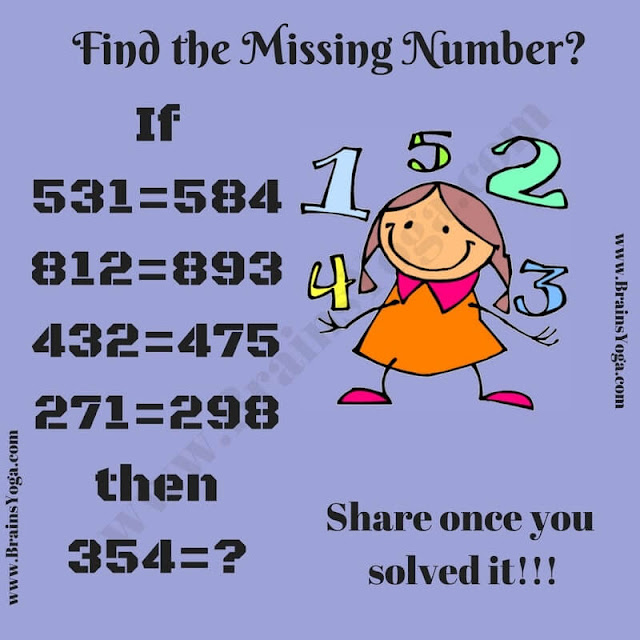Put your number game skills to the test with a maths logic puzzle. Engage in this captivating brain teaser and discover the answer.

Test your Mathematical and Logical skills by solving this picture puzzle. Can you find the logic of these given Maths equations? Once you are able to find the logical pattern in given equations, Find the number which replaces the question mark?Can you find the number which replaces the question mark?